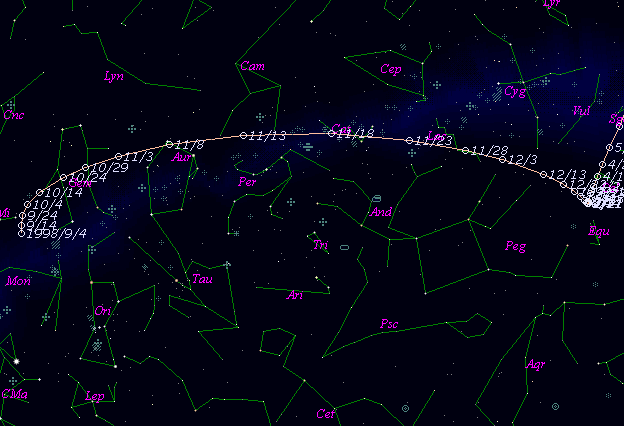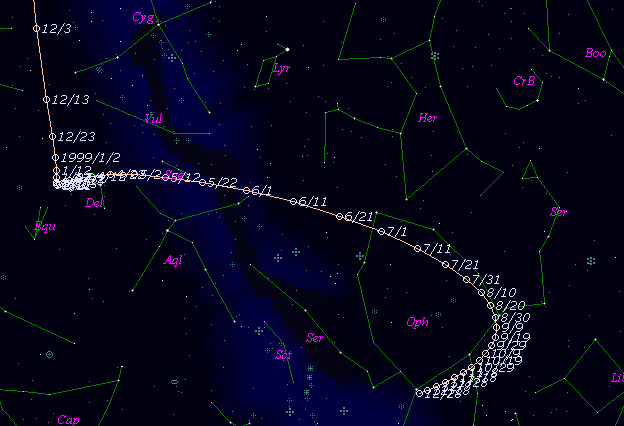# \$B%j%K%"WB@1(B

C/1998 U5 ( LINEAR )

 English version Home page Updated on September 15, 1999###\$B%W%m%U%#!<%k(B

 \$BH/8+F|(B 1998\$BG/(B10\$B7n(B30\$BF|(B \$BH/8+8wEY(B 14.0\$BEy(B \$BH/8+ Lincoln Laboratory Near-Earth Asteroid Research project

###\$B###\$B50F;MWAG(B

```   The following improved orbital elements by Kenji Muraoka, are
from 358 observations 1998 Oct. 30 to 1999 May 24, perturbations
by 9 Planets, Moon and 5 minor planets were taken into account.
The mean residual is +/- 0.68 arc seconds.

Epoch  =  1998 Dec. 13.0  TT       JDT = 2451160.5
T  =  1998 Dec. 21.76008       +/- 0.00018 (m.e.) TT
Peri. =   51.13442                +/- 0.00009
Node  =   66.65075                +/- 0.00011   (2000.0)
Incl. =  131.76490                +/- 0.00006
q  =    1.2364519              +/- 0.0000009 AU
e  =    0.9879752              +/- 0.0000075
1/a  =   +0.0097253              +/- 0.0000060 1/AU
( P  =   1043 years )
orig. 1/a  =   +0.010054
fut.  1/a  =   +0.009752
```

###\$B@1?^(B###\$B8wEYJQ2=(B

```        m1 = -1.0 + 5 log\$B&\$(B + 86   log r  [   ,-35]  (              \$B!A(B1998\$BG/(B11\$B7n(B16\$BF|(B)
m1 =  8.7 + 5 log\$B&\$(B + 10.0 log r  [-35, 50]  (1998\$BG/(B11\$B7n(B16\$BF|!A(B1999\$BG/(B 2\$B7n(B 9\$BF|(B)
m1 = 10.7 + 5 log\$B&\$(B + 10.0 log r  [ 50,   ]  (1999\$BG/(B 2\$B7n(B 9\$BF|!A(B              )
```##### \$B50F;MWAG\$OB<2,7r<#;a\$N7W;;\$K\$h\$k\$b\$N\$G\$9!#(B \$B@1?^\$O(B StellaNavigator Ver.2.0 for Windows (\$B%"%9%H%m%"!<%D(B \$BJTCx(B / \$B%"%9%-!<=PHG6I4)(B) \$B\$G:n@.\$7\$?\$b\$N\$G\$9!#(B \$B8wEY%0%i%U\$O(BComet for Windows\$B\$G:n@.\$7\$?\$b\$N\$G\$9!#(B